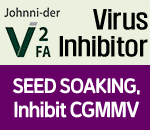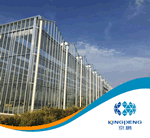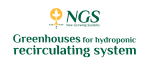# N:K ratio for phenological growth stages of net melon cultivated in NFT hydroponic system

The adequate ratio in the supply of nitrogen (N) and potassium (K) for each phenological growth stage of melon is fundamental for its growth and development with the maximization of the dry mass partition between the vegetative and reproductive parts.

The objective of a new study was to evaluate the influence of N and K concentrations in two phenological growth stages (vegetative and reproductive stage) of melon and obtain the best N:K ratio for each one.

In the first stage, four concentrations of N (8, 11, 14 and 17 mmol L-1) and two concentrations of K (4 and 5 mmol L-1) were evaluated in a randomized block design in a 4 × 2 factorial scheme with five replicates. In the second stage, in a 2 × 2 factorial scheme, the combinations of the two concentrations of N that generated the best characteristics in the previous stage and two concentrations of K (4.5 and 9.0 mmol L-1) were evaluated.

The increase of the N concentration in the vegetative stage promoted the increase of the N, P and S content of leaves and the reduction of K, Ca and Mg. Moreover, it increased the number of leaves, the height of the plant (vertically tutored) and the leaf area. Therefore, 17:5 mmol L-1 was considered as the best N:K ratio for the vegetative stage. In the second stage, there was no effect of N and K concentrations on leaf area, yield and fruit quality. So, 14:4.5 mmol L-1 was considered as the best N:K ratio for the reproductive stage.

Access the full study at ResearchGate.

Publication date: